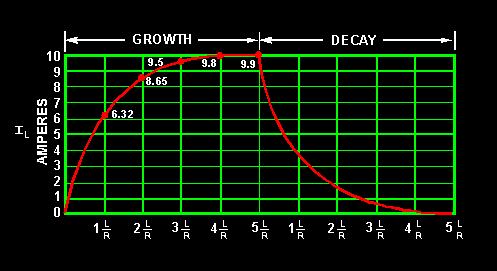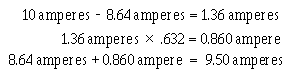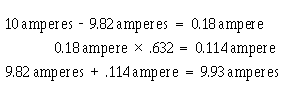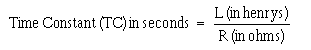L/R Time ConstantCustom SearchIn a series circuit containing a resistor (R 1) and an inductor (L1), what voltage exists across R1 when the counter emf is at its maximum value? What happens to the voltage across the resistance in an LR circuit during current buildup in the circuit, and during current decay in the circuit? L/R Time Constant The L/R TIME CONSTANT is a valuable tool for use in determining the time required for current in an inductor to reach a specific value. As shown in figure 2-11, one L/R time constant is the time required for the current in an inductor to increase to 63 percent (actually 63.2 percent) of the maximum current. Each time constant is equal to the time required for the current to increase by 63.2 percent of the difference in value between the current flowing in the inductor and the maximum current. Maximum current flows in the inductor after five L/R time constants are completed. The following example should clear up any confusion about time constants. Assume that maximum current in an LR circuit is 10 amperes. As you know, when the circuit is energized, it takes time for the current to go from zero to 10 amperes. When the first time constant is completed, the current in the circuit is equal to 63.2% of 10 amperes. Thus the amplitude of current at the end of 1 time constant is 6.32 amperes. Figure 2-11. - L/R time constant.During the second time constant, current again increases by 63.2% (.632) of the difference in value between the current flowing in the inductor and the maximum current. This difference is 10 amperes minus 6.32 amperes and equals 3.68 amperes; 63.2% of 3.68 amperes is 2.32 amperes. This increase in current during the second time constant is added to that of the first time constant. Thus, upon completion of the second time constant, the amount of current in the LR circuit is 6.32 amperes + 2.32 amperes = 8.64 amperes. During the third constant, current again increases:During the fourth time constant, current again increases:During the fifth time constant, current increases as before:Thus, the current at the end of the fifth time constant is almost equal to 10.0 amperes, the maximum current. For all practical purposes the slight difference in value can be ignored. When an LR circuit is deenergized, the circuit current decreases (decays) to zero in five time constants at the same rate that it previously increased. If the growth and decay of current in an LR circuit are plotted on a graph, the curve appears as shown in figure 2-11.Notice that current increases and decays at the same rate in five time constants. The value of the time constant in seconds is equal to the inductance in henrys divided by the circuit resistance in ohms. The formula used to calculate one L/R time constant is:Q.9 What is the formula for one L/R time constant? Q.10 The maximum current applied to an inductor is 1.8 amperes. How much current flowed in the inductor 3 time constants after the circuit was first energized?Integrated Publishing, Inc. - A (SDVOSB) Service Disabled Veteran Owned Small Business